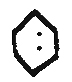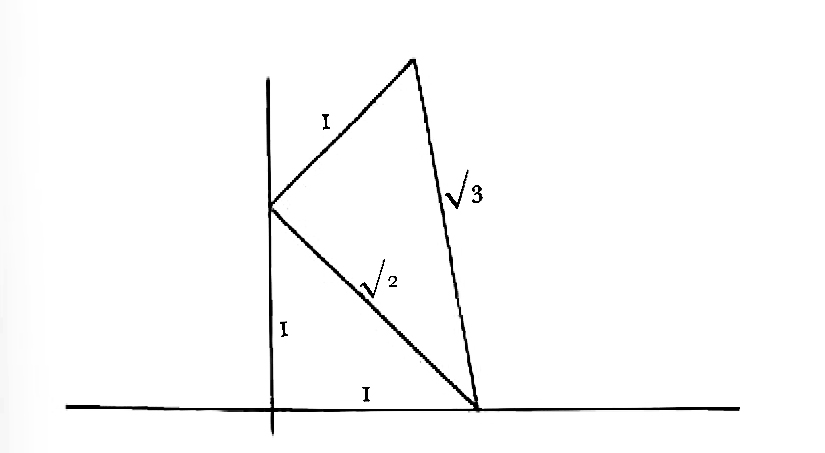# From E. A. Darwin   [before 8 June 1858]1

Dear Charles.

To make sure that I now understand look at my diagram.2 The twoA, F in the hexagon the section of the Dodec.

Call CB radius of sphere (r) & CF radius of ⊙ in which the hexagon is inscribed r′, then

 r′ : r :: .8165 : 1 in the bees hexagon r′ = 0inch.125 or ⅛ inch .:. r = $\frac{r\prime }{.8165}$ = $\frac{.125}{.8165}$ = 0inch.153 r−r′ = 0inch.028 distance sought. The distance from center to obtuse ∠ = r .866 = 0in.153 ×.866 = 0in.1325 distance to ob: ∠ of Dodec = 0.1325 r′ = distance to ∠ of Heg = 0.1250 Difference = 0.0075 or ¾ of $\frac{1}{100}$ inch.

Make no scruple of asking any number of explanations, as it is very little trouble to me, & saves you bother.3

Yours E D

## [Enclosure]4

 CB = R = 1CC’= R $\sqrt{2}$ = 1.414 EF = $\frac{1}{2}$ FG = side of hexagon EF = EC × tang 30o = EC × $\frac{1}{\sqrt{3}}$ EC = $\frac{\sqrt{2}}{2}$ 2EF = FG = $\frac{\sqrt{2}}{\sqrt{3}}$ = .8165## CD annotations

Bottom of first page: ‘0.028 0.056 0.25 0.306 = diameter of sphere’; ‘sphere rather more than $\frac{1}{20}$ inch larger than hexagon’ pencil . Enclosure: ‘or one side of hexagon = radius’; ‘One side of Hexagon in circle equals radius of circle.’ pencil \note5
Erasmus says Dodecahedron diameter is radius 2. In Wasps nest, hexagon = radius of sphere (on hexagonal theory) & saucer up to lower part of festoon will equal half radius.— from angle to angle of hexagon of course = twice radius. [therefore sign] saucer $\frac{1}{4}$ of diameter of hexagon.—

## Footnotes

Dated by the relationship to the following letter.
See enclosure.
CD was eager to revise his calculations relating to the construction of bees’ cells (see letter to W. E. Darwin, [26 May 1858]). Erasmus Alvey Darwin had helped him in the past with geometrical problems (see letter from E. A. Darwin, [May–June 1858], and Correspondence vol. 3, letter from E. A. Darwin, [May 1844 – 1 October 1846]).
The enclosure is in DAR 48 (ser. 2): 46 in association with CD’s notes on bees’ cells.
The note is in DAR 162: 48/2.

## Bibliography

Correspondence: The correspondence of Charles Darwin. Edited by Frederick Burkhardt et al. 29 vols to date. Cambridge: Cambridge University Press. 1985–.

## Summary

Gives calculations on the structure of bees’ cells.

## Letter details

Letter no.
DCP-LETT-2278
From
Erasmus Alvey Darwin
To
Charles Robert Darwin
Sent from
unstated
Source of text
DAR 162: 48b
Physical description
ALS 2pp †, CD note